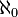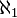# Cardinal number facts for kids

Kids Encyclopedia Facts
(Redirected from Cardinal numbers)

Cardinal numbers (or cardinals) are numbers that say how many of something there are, for example: one, two, three, four, five, six. They are sometimes called counting numbers.

The cardinality of a set is the cardinal number that tells us, roughly speaking, the size of the set.

In mathematics, people also study infinite cardinal numbers. The first infinite cardinal number was named$\aleph_0$ (pronounced "Aleph null", "Aleph-zero" or "Aleph-naught") by Georg Cantor.$\aleph_0$ is the number of numbers that are in the group 0, 1, 2, 3, ... (which goes on forever). Both the set of natural numbers and the set of rational numbers are of size$\aleph_0$ (that is, they are both countable).

Another infinite cardinal number is the number of numbers in the set of real numbers, and is represented by the symbol$\aleph_1$ (or$\mathfrak{c}$, the cardinality of the continuum). Cantor proved that there are many different infinite cardinal numbers that are bigger than$\aleph_0$. A famous theorem of Cantor is that the cardinality of the real numbers is larger than the cardinality of the natural numbers. The continuum hypothesis is the statement that there is no middle cardinal number strictly between that of natural numbers and real numbers.

## Related pagesCardinal number Facts for Kids. Kiddle Encyclopedia.